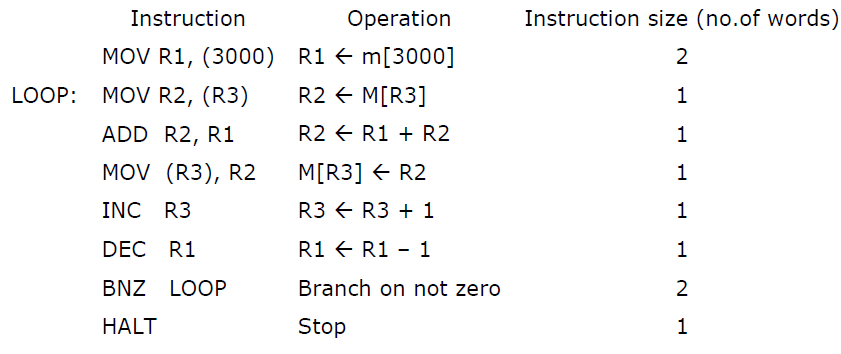Related Articles

# GATE | GATE-CS-2007 | Question 71

• Last Updated : 19 Nov, 2018

Consider the following program segment. Here R1, R2 and R3 are the general purpose registers.Assume that the content of memory location 3000 is 10 and the content of the register R3 is 2000. The content of each of the memory locations from 2000 to 2010 is 100. The program is loaded from the memory location 1000. All the numbers are in decimal. Assume that the memory is word addressable. The number of memory references for accessing the data in executing the program completely is:
(A) 10
(B) 11
(C) 20
(D) 21

Explanation: Explanation:

Ist memory reference R1←M and then in the loop which runs for 10 times, because the content of memory location 3000 is 10 given in question and loop will run 10 times as

R2← M[R3]

M[R3] ←R2

There are two memory reference every iteration

10*2=20

Total=20+1=21

So  (D) is correct option.

Attention reader! Don’t stop learning now.  Practice GATE exam well before the actual exam with the subject-wise and overall quizzes available in GATE Test Series Course.

Learn all GATE CS concepts with Free Live Classes on our youtube channel.

My Personal Notes arrow_drop_up